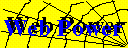# Power Analysis FAQ

Just a beginning....
Are the sample sizes calculated for each group or for the whole design?
Short answer: In general, FPOWER calculates power power and sample size for each level of the effect designated 'A' in the design.
Does this program (FPOWER) handle repeated measure designs?
Short answer: FPOWER calculates power only for between-subject effects.

Long answer: The FPOWER macro computes power of an F-test for main effects or interactions for between-subject effects in factorial designs with or without repeated measures, assuming main effects are fixed. That is, the design may include repeated measure factors, but the program cannot calculate power for those effects.

The program can be used for ANY fixed effect in ANY crossed factorial design, by designating the levels of the effect of interest as A, and the levels of all other crossed factors as B.

It does *not* (correctly) handle power for repeated-measure factors, split-plot designs, etc, for which power analysis is much more complex.

What formula should I use to calculate RHO (intra class correlation) for a repeated measure design?
This is usually an estimate (rather than a calculated value) of the average correlation between repeated measurements.
Is there a way to calculate power for MANOVA designs?
There's a SAS macro, mpower.sas, which carries out retrospective power analysis for between-subject MANOVA designs. See MPOWER.

For more complex MANOVA designs, Muller, LaVange, Ramey, and Ramey (Journal of the American Statistical Association, 1992, p1209-1226) provide a survey of power for multivariate models. A SAS/IML program which carries out many of these analyses, including repeated measures designs is available at the SAS File Contribution Server at ftp://ftp.uga.edu/pub/sas/contrib/cntb0014. To use it, you must thoroughly understand the General Linear Model and be able to specify your hypotheses in matrix form.

I am not aware of other software which handles MANOVA designs.